## Ordinary Differential Equation--First-Order Exact

Consider a first-order ODE in the slightly different form(1)

Such an equation is said to be exact if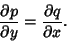(2)

This statement is equivalent to the requirement that a Conservative Field exists, so that a scalar potential can be defined. For an exact equation, the solution is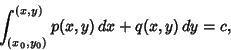(3)

whereis a constant.

A first-order ODE (1) is said to be inexact if(4)

For a nonexact equation, the solution may be obtained by defining an Integrating Factorof (6) so that the new equation(5)

satisfies(6)

or, written out explicitly,(7)

This transforms the nonexact equation into an exact one. Solving (7) forgives(8)

Therefore, if a functionsatisfying (8) can be found, then writing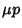(9)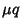(10)

in equation (5) then gives(11)

which is then an exact ODE. Special cases in whichcan be found include-dependent,-dependent, and-dependent integrating factors.

Given an inexact first-order ODE, we can also look for an Integrating Factorso that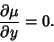(12)

For the equation to be exact inand, the equation for a first-order nonexact ODE(13)

becomes(14)

Solving forgives(15)

which will be integrable if(16)

in which case(17)

so that the equation is integrable(18)

and the equation(19)

with knownis now exact and can be solved as an exact ODE.

Given in an exact first-order ODE, look for an Integrating Factor. Then(20)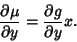(21)

Combining these two,(22)

For the equation to be exact inand, the equation for a first-order nonexact ODE(23)

becomes(24)

Therefore,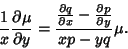(25)

Define a new variable(26)

then, so(27)

Now, if(28)

then(29)

so that(30)

and the equation(31)

is now exact and can be solved as an exact ODE.

Given an inexact first-order ODE, assume there exists an integrating factor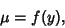(32)

so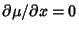. For the equation to be exact inand, equation (7) becomes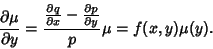(33)

Now, if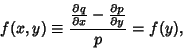(34)

then(35)

so that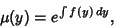(36)

and the equation(37)

is now exact and can be solved as an exact ODE.

Given a first-order ODE of the form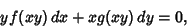(38)

define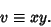(39)

Then the solution is(40)

If(41)

where(42)

then letting(43)

gives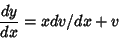(44)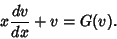(45)

This can be integrated by quadratures, so(46)(47)

References

Boyce, W. E. and DiPrima, R. C. Elementary Differential Equations and Boundary Value Problems, 4th ed. New York: Wiley, 1986.